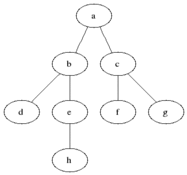# 广度优先搜索 BFS算法

## 算法思想

1、首先将根节点放入队列中。

2、从队列中取出第一个节点，并检验它是否为目标。

• 如果找到目标，则结束搜索并回传结果。
• 否则将它所有尚未检验过的直接子节点加入队列中。

3、若队列为空，表示整张图都检查过了——亦即图中没有欲搜索的目标。结束搜索并回传“找不到目标”。

4、重复步骤2。

## 搜索过程演示#### 说明

• 灰色的是加入队列中的活动节点，黑色是从活动节点队列中选取的扩展节点。

• 每次将一个活动节点标记为扩展节点后，进行判断，并将该点从活动节点队列中移除，再将该节点所有未检测的子节点加入活动节点队列中去。

• 接下来再从队列中选取新的活动节点作为扩展节点，如此循环下去，直至队列为空为止，说明已经遍历过所有的节点。

## 复杂度

• 因为所有节点都必须被存储，因此BFS的空间复杂度为 O(|V|+|E|)，其中 |V| 是节点的数目，而 |E| 是图中边的数目。

• 最差情形下，BFS必须查找所有到可能节点的所有路径，因此其时间复杂度为 O(|V|+|E|)。

## C++实现

//Given a binary tree, find its minimum depth.
//The minimum depth is the number of nodes along
//the shortest path from the root node down to
//the nearest leaf node.

// 递归方法
class Solution
{

public:
int run(TreeNode *root)
{
if (!root)
{
return 0;
}

// 若左子树为空，返回右子树深度 + 1
if (root->left == nullptr)
{
return (run(root->right) + 1);
}
// 若右子树为空，返回左子树深度 + 1
if (root->right == nullptr)
{
return (run(root->left) + 1);
}

// 左右子树都不为空，返回较小值
int leftDepth = run(root->left);
int rightDepth = run(root->right);
return ((leftDepth < rightDepth) ? (leftDepth + 1) : (rightDepth + 1));

}
};

// BFS方法

#include <vector>
using namespace std;
class Solution
{
public:
int run(TreeNode *root)
{
if (!root)
{
return 0;
}

vector <TreeNode *> que;
TreeNode *now = root;  // 当前访问的节点
TreeNode *last = root;  // 每层最后的一个节点
que.push_back(root);
int depth = 1;  // 初始深度

while (que.size())
{
// 取出队列中的第一个节点作为当前节点
now = que.front();
que.erase(que.begin());

// 如果当前节点没有子节点了，直接终止循环，说明是叶子节点，返回最小深度
if ((now->left == nullptr) && (now->right == nullptr))
{
break;
}

// 将子节点加入队列中
if (now->left != nullptr)
{
que.push_back(now->left);
}
if (now->right != nullptr)
{
que.push_back(now->right);
}

// 当访问到每层的最后一个节点时，深度+1
if (now == last)
{
depth++;
last = que.back();  // 将下一层最后一个节点赋给last
}

}

return depth;

}

};


## BFS在迷宫问题的应用

• #### MTK编程挑战赛决赛《算术迷宫》

posted @ 2018-08-02 15:16  东聃  阅读(...)  评论(... 编辑 收藏